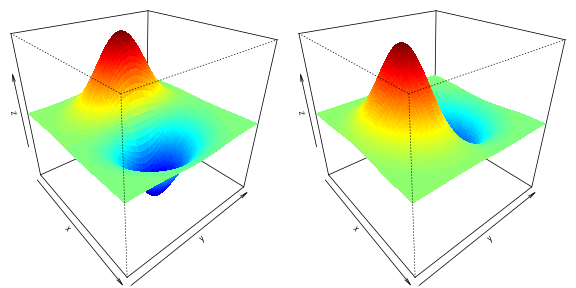## First Derivative of the Multivariate Normal Densities with RcppArmadillo

Joscha Legewie — written Sep 9, 2013 — source

There is a great RcppArmadillo implementation of multivariate normal densities. But I was looking for the first derivative of the multivariate normal densities. Good implementations are surprisingly hard to come by. I wasn’t able to find any online and my first R implementations were pretty slow. RcppArmadillo might be a great alternative particularly because I am not aware of any c or Fortran implementations in R. In these areas, we can expect the largest performance gains. Indeed, the RcppArmadillo version is over 400-times faster than the R implementation!

First, let’s take a look at the density function $x \sim N(m,\Sigma)$ as shown in the The Matrix Cookbook (Nov 15, 2012 version) formula 346 and 347

where $\mathbf{x}$ and $\mathbf{m}$ are d-dimensional and $\mathbf{\Sigma}$ is a $d \times d$ variance-covariance matrix.

Now we can start with a R implementation of the first derivative of the multivariate normal distribution. First, let’s load some R packages:

Here are two implementations. One is pure R and one is based on dmvnorm in the mvtnorm package.

These implementations work but they are not very fast. So let’s look at a RcppArmadillo version. The Mahalanobis function and the first part of dmvnorm_deriv_arma are based on this gallery example, which implements a fast multivariate normal density with RcppArmadillo.

Now we can compare the different implementations using simulated data.

                                    test replications elapsed relative
1            dmvnorm_deriv_arma(X, m, s)           10   0.021    1.000
3 dmvnorm_deriv2(X, mean = m, sigma = s)           10   6.813  324.429
2   dmvnorm_deriv1(X, mu = m, sigma = s)           10  22.439 1068.524


The RcppArmadillo implementation is several hundred times faster! Such stunning performance increases are possible when existing implementation rely on pure R (or, as in dmvnorm_deriv2, do some of the heavy lifting in R). Of course, the R implementation can probably be improved.

Finally, let’s plot the x- and y- derivates of the 2-dimensional normal density function.Note: This is an updated version dated 2015-01-06 of the original article.